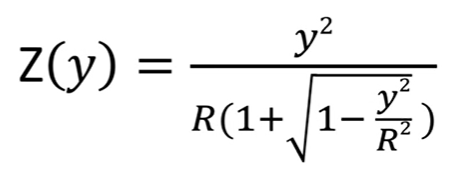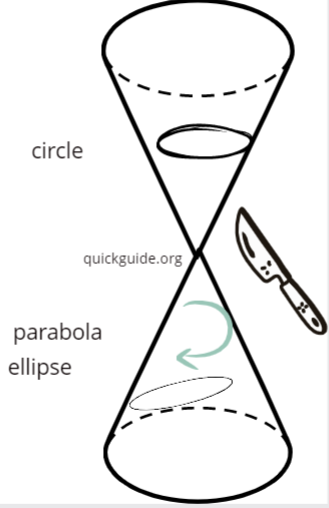top of page
Search

# Spheric, Aspheric and Freeform lenses

The objective of this article is to understand different types of lenses and their effect on spherical aberration correction. We understand that spherical aberration occurs when marginal rays of light are over refracted than the paraxial rays of light. The amount of spherical aberration that occurs while marginal rays pass through the lens, will depend on the lens shape in itself. For those of us who are connected to ophthalmology, a spherical lens design almost always connects to a biconvex shape. This is because all IOLs are shaped biconvex. But in effect, a spherical lens could be a plano convex, a convex plano, or even a meniscus shaped lens.Source: Wikipedia

Thus all spherical lenses, be it a biconvex or a plano convex, or otherwise would give rise to spherical aberration. Spherical aberration cannot be totally eliminated by spherical lenses, although its effect can be minimized with a spherical lens of an appropriate bending of light. This is also called the Coddington Shape Factor. Another factor that also determines the amount of spherical aberration that happens through a particular spherical lens is the position of the image and object distances, also called the Coddington Position Factor. Combining the two, would help us to achieve the least spherical aberration with a spherical lens.

C (coddington shape factor) = r2 +r1/r2 - r1 ----- (1)

( wherein the r2 and r1 are the two radius of curvature of the anterior and posterior of the lens)

P (coddington position factor) = i + o/i- o ------- (2)

( where i and o are image and object distances)

Considering Fig 1, the minimum spherical aberration that could be achieved through IOLs, appear to be a shape factor between 1 and 0 when the object is at infiniti.Fig 1 : Shape factor and spherical aberration. Source : O. Faruk Farsakoglu* a , Ipek Inal Atik a , Hikmet Kocabasb

However, one other conclusion that we can draw from Fig 1 is that regardless of the design of the spherical lens, that is the shape factor, the spherical aberration cannot be altogether eliminated with such lenses. And herein comes the importance of aspheric lenses. But before we describe aspheric lenses, let us look into the spherical lens surface in Fig 2, so that it would be easier then to understand different aspheric lenses when we further go ahead.

Spherical lenses - Spherical lenses are a cross section of a circle. A circle can be defined as a set of points from the same plane which are having an equal distance from a centre point. Thus a line that joins the centre of the circle to the edge of lens is the radius (r). The radius of curvature (R) is constant across the surface of the sphere, therefore the surface contour of the lens follows a regular arc where the lens surface is always the same distance away from the centre of curvature (centre point).Fig 2 : spherical surface quickguide.org

The Z(y) represents the deviation of the surface of the sphere from the tangent drawn as a straight line on the vertex of the lens. The 'y' is the distance from the vertex of the lens to the saggital (sag) point being measured. By finding the Z(y), that is the sag, we can understand the surface of the sphere or construct the sphere.

The equation therefore for a spherical surface is :It is not about memorizing this formulae, but understanding the three components of the formulae, that is the 'y' is the distance from the vertex where the consecutive sagittal, Z(y) is being measured as a function of the R (radius of curvature of circle). With this formulae therefore you can calculate the full optical surface of the sphere.

Conic section

Like the circle, there are other shapes like elipse, parabola, hyperbola, etc. The earth's orbit around the sun is an elipse. On the other hand the shape of a parabola and hyperbola is described in Fig 3. In a parabola, all rays of light passing through the lens surface will come and meet at the same point. Thus, the mirror of a torch light, or a dish of the satellite is shaped like a parabola. Since a parabola has the property to focus all rays in a single point, therefore satellite dish is made in the shape of parabola. Thus all rays that reflect off the dish of the satellite are focussed on the receiver. A human cornea that is shaped like a parabola has no spherical aberration. All such shapes come under one category and is called conics as they can be cut out from a cone, that is they are all part of cross section of a cone. That is why they are called conic section.The shape of the conic section, that is elipse/circle/parabola, etc can be described by its Konic constant or kappa, denoted by 'K'. The radius of curvatue is varying, and therefore is described by the K. When K is equal to zero, the shape derived from the cone is that of a circle/sphere (Fig 4)Aspherics - Any deviation from a spherical surface may be defined as aspheric. Thus when K is zero, the shape is spherical, and therefore for all other shapes generated from the conic constant, the shapes are aspheric. The conic constant (K) of a parabola is -1.

The nice thing of all these conic sections (including parabola, the thing of our interest) is that you can describe the surface just by taking into account the radius of curvature and its conic constant.Fig 5 - Parabola surface

So thus, while in the previous section we had described the formula for a sphere ( conic constant 0 ), here is the expression for an aspheric lensWhere K is the conic constant ( take the value as -1 and you arrive at a parabola with no spherical aberration), R the radius of curvature, y the distance from the vertex, and z(y) is the saggital or deviation from a base sphere. Basically, using the vertex (center) of the lens as a starting point, the equation tells us how much the lens surface deviates or "sags" along the Z axis (up or down) at any given distance “y” from the center.

Basically, using the vertex (center) of the lens as a starting point, the equation tells us how much the lens surface deviates or "sags" along the Z axis (up or down) at any given distance “y” from the center when plotted perpendicularly to the optical axis.

Thus we can arrive at the construction of a standard aspheric surface, be it a parabola ( where K is -1) or a hyperbola, or any other asphere.

Freeform even order aspherics

Not every shape can be described by a conic section alone. We know that aspheric lenses will have a variable radius of curvature. But the standard aspheric lens would have either a convex shape or a concave shape ( in ophthalmology we talk about convex aspherics generally). However, in a freeform aspheric, there would be areas of concavity in an otherwise convex lens. The point where the lens turns from a convex shape to a concave shape is called its inflection point (Fig 6 shows inflection points in a freeform lens). Thus Inflection Point is a point on the asphere where the local radius changes sign, e.g., from a convex to a concave radius. Such a lens is more difficult to manufacture and is complex.Fig 6 - Freeform surface. Inflection points are shown

While a standard aspheric lens formulae has been described above, the freeform lens because of its complexities (inflection points) cannot be described by a standard polynomial function and therefore needs to be described by other higher order polynomial terms to correctly describe or construct the surface shape. Below in the equation the standard aspheric lens equation and the higher order polynomial is described.Thus if you see that a lens is described by the above equation, you will know that it is a standard aspheric lens (conic constant as defined by company) with local radius of curvature changes from convex to concavity (inflection points). In the above equation, the part described as higer order polynomial function has terms of y that describes the deviation from the conical shape (conic constant) from the vertex of the lens. Each of the higher order terms (y) as a coefficient alpha that indicate how much each term contribute to the shape and in this particular formula the powers of y are limited to even numbers, and hence even orders ( 2, 4, 6, etc ). Thus the even order terms of y indicate the local radius of curvature change at those inflection points.

To have a closer look into what is an even order polynomial function in an aspheric lens and how it is different than an odd order, consider figure 7. The even and odd order is with respect to the y-axis. In the left graph, both hands rise symmetric to its y-axis while on the right while one hand is pointing above the other below. In an even order polynomial, both hands go in the same direction, while in the odd one hand goes up and the other down.Figure 7 Even order and odd order polynomials

One of the reasons why freeform optics is so attractive is that it allows designers to simultaneously correct aberrations while increasing Depth of Field and field of view. Depth of field is an important aspect and is finding new interest in IOL science, while field of view is and equally interesting subject in designing other types of lenses, mainly the spectacles. Freeform lenses, also called digital high definition glasses can surface lenses in power increments of .01 diopter, compared to conventional lenses that can achieve precision only upto .25 diopters.

---------------------------------------------------------------------------------------------------------------------------

Just in case

Polynomial function - Poly means many and nomial means terms. A polynomial can be an expression with one term ( 5x), two terms ( 5y + 4z) or three terms ( 14x +15y -3z). More than three term are usually referred to as higher order terms. This higher order term is a feature of a freeform lenses.

-----------------------------------------------------------------------------------------------

References:

• A correlation of thin lens approximation to thick lens design by using context based method in optics education O. Faruk Farsakoglu* a , Ipek Inal Atik a , Hikmet Kocabasb. ETOP 2013

435 views

See All
bottom of page